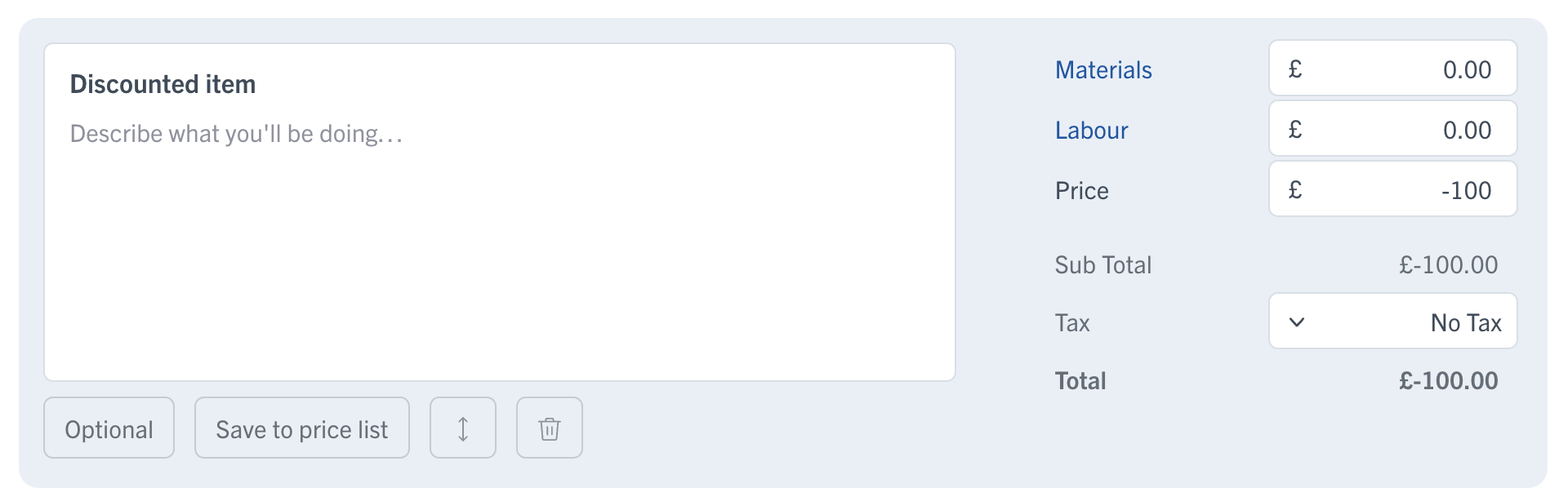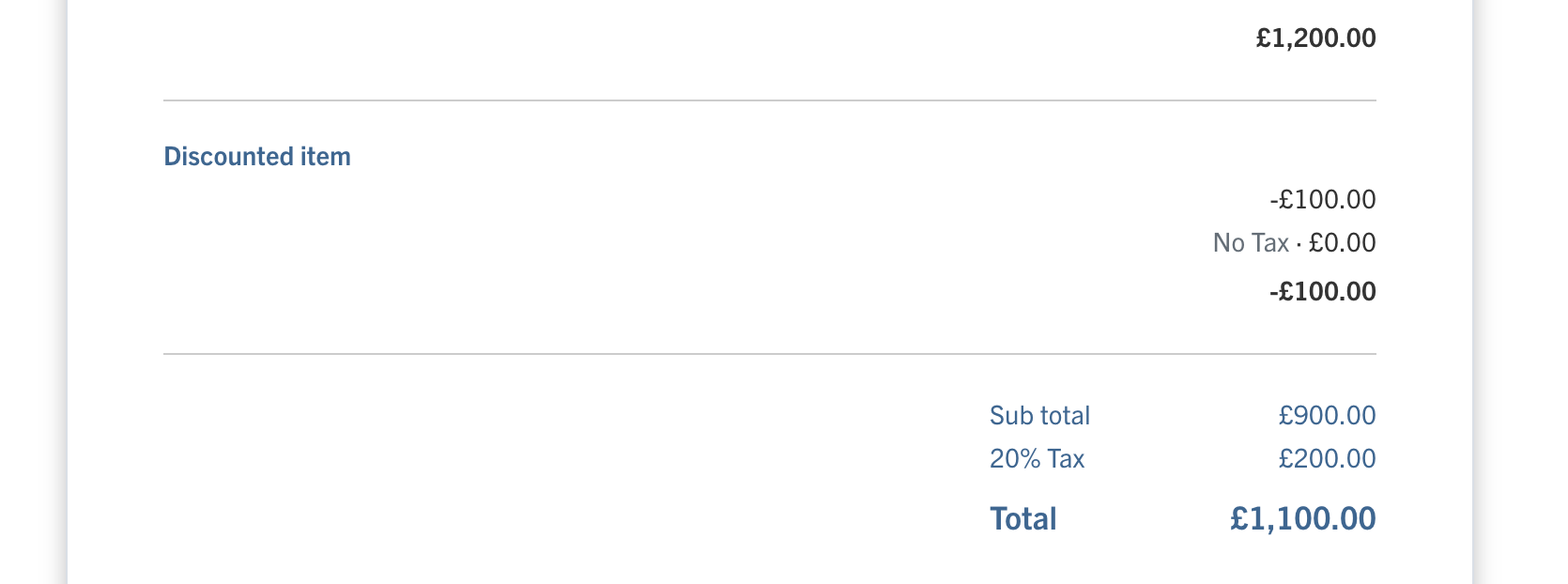# Adding a discount percentage (%)

Discount percentages can be applied to the paperwork total paperwork.

Here's how:

1. Within the paperwork totals, select + Add Discount
2.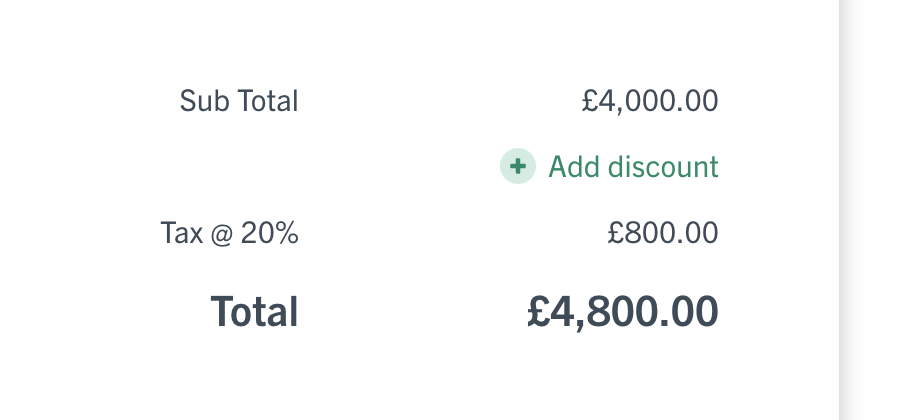Choose the percentage discount you'd like to apply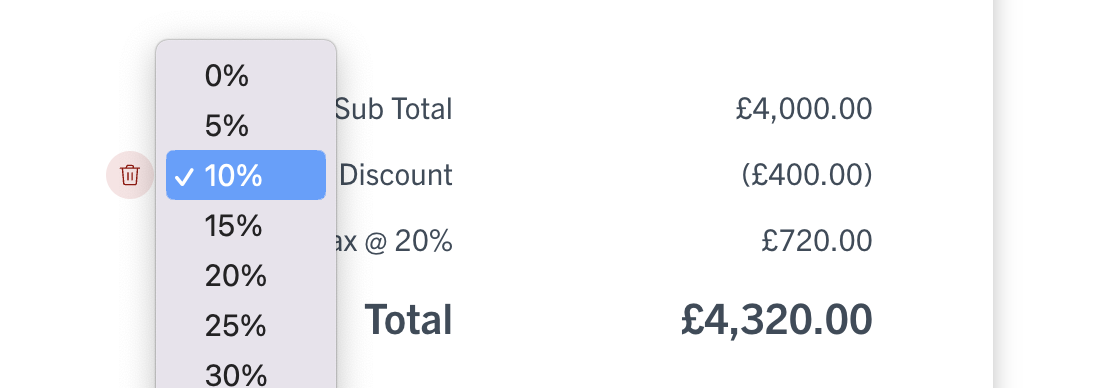3. 🎉 That's it! Each paperwork item now includes the discounted amount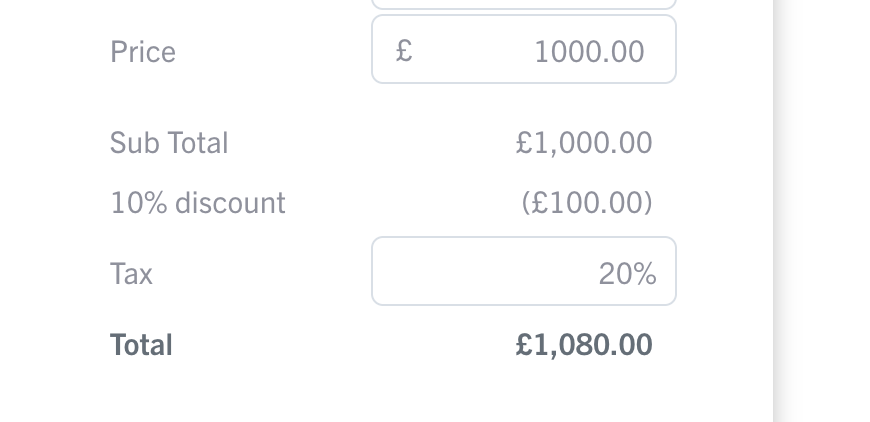HINT: You can enter a different percentage amount by selecting the "Other…" option.

To remove a percentage discount, simply select the trash can icon. Your item totals will then be re-calculated without the discount.

Discount amounts can be applied by adding a new work item with a negative price.

Here's how: